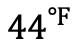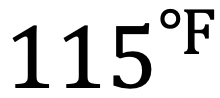## How to Calculate Z-Score?: Statistics

Hi there and welcome to MathSux! In this post, we are going to explore how to calculate z-score and the normal distribution. We’ll do this by examining the normal curve and learning how to find probability finding z-score and using the mean, standard deviation, and specific data points. Fore more info and more MathSix don’t forget to check out the video and practice questions below. Happy calculating!

## What is a Normal Curve?

A normal curve is a bell shaped curve that shows the distribution of data evenly spread with respect to the mean. If you look at the normal curve below, the area under the curve shows all the possible probabilities of a certain data point occurring, notice the curve is higher towards the center mean, μ, and gets smaller as the distance from μ grows. The distance from μ is measured by the standard deviation, a unique unit of measurement that is specific to each group of data.

Mean: The mean always falls directly in the center of our normal curve. It is the average of our data, and always falls right in the middle.

Standard Deviation:  This value is used as a standard unit of measurement for the data, measuring the distance between each data point in relation to the mean throughout the entire data set. For a review on what standard deviation is and how to calculate it, check out this post here.

Now for our normal curve:

Notice half of the data is below the mean, μ, while the other half is above? The normal curve is symmetrical about the mean, μ!

## How to Calculate Z-Score?

Z Score can tell us at what percentile a certain point in the data set falls in relation to the rest of the mean by using the standard deviation as a unit of measurement.  If this sounds confusing, it’s ok! Take a look at the following formula:

We use the above formula in conjunction with  a z table which tells us the probability under the curve for a certain point.

## a) What percent of student scored below 500?

Step 1: First, let’s draw out our given information the mean=500, standard deviation=100, and the data point the question is asking for x=500 onto a normal curve. Notice that we want to find the value of the area under the curve shaded in pink.  This will tell us the percent of students that scored below 500.

Step 2: We need to find the z-score by, using the data point given to us x=500, the mean=500, and the standard deviation, sigma=100.

Step 3: Yes, we have a zero! Now we need to take our z table and line up our chart. Notice that the chart finds the probability for everything at the beginning of the normal curve and on.  This is perfect for answering our question!

Step 4: The table gives us our solution of .5000.  If we multiply .5000 times 100 it gives us the percent of students who scored below 500 at 50%.

## b) What percent of student scored above 620?

Step 1: First, let’s draw out our given information the mean=500, standard deviation=100, and the data point the question is asking for x=620 onto a normal curve. Notice that we want to find the value of the area under the curve shaded in pink.  This will tell us the percent of students that scored above 620.

Step 2: We need to find the z-score by, using the data point given to us x=620, the mean=500, and the standard deviation, sigma=100.

Step 3: Yes, we got 1.2! Now we need to take our z table and line up our chart. Notice that the chart finds the probability for everything at the beginning of the normal curve and on.  This is means to find the percent we are looking for, we need to subtract our answer from one since we want the value of probability on the right side of the curve (the z-table only provides the left side).

Step 4: The table gives us our solution of .8849.  If we subtract this value from 1 then multiply that value times 100 it gives us the percent of students who scored above 620.

## C) What is the highest score a student could receive if the students was in the 16.11th percentile?

Step 1: In this question we have to work backwards by first identifying, where on the z-score table is the number .1611 and then filling in our z score formula to find x, the missing data point (in this case test score).

Search the table for .1611:

Notice that .1611 can be found on the z-table above with z-score -0.99.  This is what we’ll use to find the unknown data point!

Step 2: We need to find the unknown test score by, using the z score we just found z=-0.99, the mean=500, and the standard deviation, sigma=100.

Step 3: Solve for x.

## Practice Questions:

The grades on a final English exam are normally distributed with a mean of 75 and a standard deviation of 10.

a) What percent of students scored below a 60?

b) What percent of students scored above an 89?

c) What is the highest possible grade that included in the 4.46th percentile?

d) What percent of students got at least a 77?

## Solutions:

a) 6.68%

b) 8.08%

c) 58

d) 42.07%

Want to make math suck just a little bit less? Subscribe to my Youtube channel for free math videos every week! 🙂

## TikTok Math Video Compilations

Happy December everyone! With crazy 2020 coming to an end, I thought I would share some TikTok math video compilations of Algebra, Geometry, Algebra 2/Trig, and Statistics for a quick review of all our videos posted throughout the year. Enjoy these TikTok math video compilations and happy calculating! 🙂

Want to make math suck just a little bit less? Subscribe and follow us for FREE fun colorful math videos and lessons every week! 🙂

## Algebra:

Within algebra, you will find arithmetic sequences, combining like terms, box and whisker plots, geometric sequences, solving radical equations, completing the square, 4 ways to factor quadratic equations, piecewise functions and more!

## Geometry:

Within Geometry, you will find, how to construct an equilateral triangle, a median of a trapezoid, area of a sector, how to find perpendicular and parallel lines through a given point, SOH CAH TOA right triangle trigonometry, reflections, and more!

## Algebra 2/Trig.

Within Algebra 2/Trig., you will find, how to expand a cubed binomial, how to divide polynomials, how to solve log equations, imaginary numbers, synthetic division, unit circle basics, how to graph y=sin(x), and more!

## Statistics:

Within statistics, you will find, box and whisker plots, how to find the variance, and, the probability of flipping a coin 2 times!

For full length video, don’t forget to check out our free math video index page! Thanks for stopping by! 🙂

## Variance and Standard Deviation: Statistics

Greetings math friends! In this post we’re going to go over variance and standard deviation. We will take this step by step to and explain the significance each have when it comes to a set of data. Get your calculators ready because this step by step although not hard, will take some serious number crunching! Check out the video below to see how to check your work using a calculator and happy calculating! 🙂

What is the Variance?

The variance represents the spread of data or distance each data point is from the mean.  When we have multiple observations in our data, we want to know how far each unit of data is from the mean.  Are all the data points close together or spread far apart?  This is what the variance tells us!

Don’t freak out but here’s the formula for variance, notated as sigma squared:

This translates to:

Let’s try an example:

What is Standard Deviation?

Standard deviation is a unit of measurement that is unique to each data set and is used to measure the spread of data. The formula for standard deviation happens to be very similar to the variance formula!

Below is the formula for standard deviation, notated as sigma:

Since this is the same exact formula as variance with a square root, all we need to do is take the square root of the variance to find standard deviation:

Now try calculating these statistics on your own with the following practice problems!

Practice Questions:

Solutions:

Still got questions? No problem! Don’t hesitate to comment with any questions or check out the video above. Happy calculating! 🙂

Also! If you’re looking for more statistics, check out this post on how to create and analyze box and whisker plots here!

## Box and Whisker Plots, IQR and Outliers: Statistics

Ahoy math friends! This post takes a look at one method of analyzing data; box and whisker plots. Box and whisker plots are great for visually identifying outliers and the overall spread of numbers in a data set. We will go over step by step how to create a box and whisker plot given a set of data, we will then look at how to find the interquartile range and upper and lower outliers. If you have any questions, don’t hesitate to check out the video or comment below. Stay curious and happy calculating! 🙂

Looking for more MathSux? Check out this post on variance and standard deviation here!

Box plots look something like this:

Why Box Plots?

Box Plots are a great way to visually see the distribution of a set of data.  For example, if we wanted to visualize the wide range of temperatures found in a day in NYC, we would get all of our data (temperatures for the day), and once a box plot was made, we could easily identify the highest and lowest temperatures in relation to its median (median: aka middle number).

From looking at a Box Plot we can also quickly find the Interquartile Range and upper and lower Outliers. Don’t worry,  we’ll go over each of these later, but first, let’s construct our Box Plot!

->  First, we want to put all of our temperatures in order from smallest to largest.
-> Now we can find Quartile 1 (Q1), Quartile 2 (Q2) (which is also the median), and Quartile 3 (Q3).  We do this by splitting the data into sections and finding the middle value of each section.

Q1=Median of first half of data

Q2=Median of entire data set

Q3=Median of second half of data

-> Now that we have all of our quartiles, we can make our Box Plot! Something we also have to take notice of, is the minimum and maximum values of our data, which are 65 and 92 respectively. Let’s lay out all of our data below and then build our box plot:

Now that we have our Box Plot, we can easily find the Interquartile Range and upper/lower Outliers.

->The Interquartile Range is the difference between Q3 and Q1. Since we know both of these values, this should be easy!

Next, we calculate the upper/lower Outliers.

-> The Upper/Lower Outliers are extreme data points that can skew the data affecting the distribution and our impression of the numbers. To see if there are any outliers in our data we use the following formulas for extreme data points below and above the central data points.

*These numbers tell us if there are any data points below 44.75 or above 114.75, these temperatures would be considered outliers, ultimately skewing our data. For example, if we had a temperature oforthese would both be considered outliers.

Practice Questions:

Solutions:

Still got questions? No problem! Don’t hesitate to comment with any questions or check out the video above. Happy calculating! 🙂